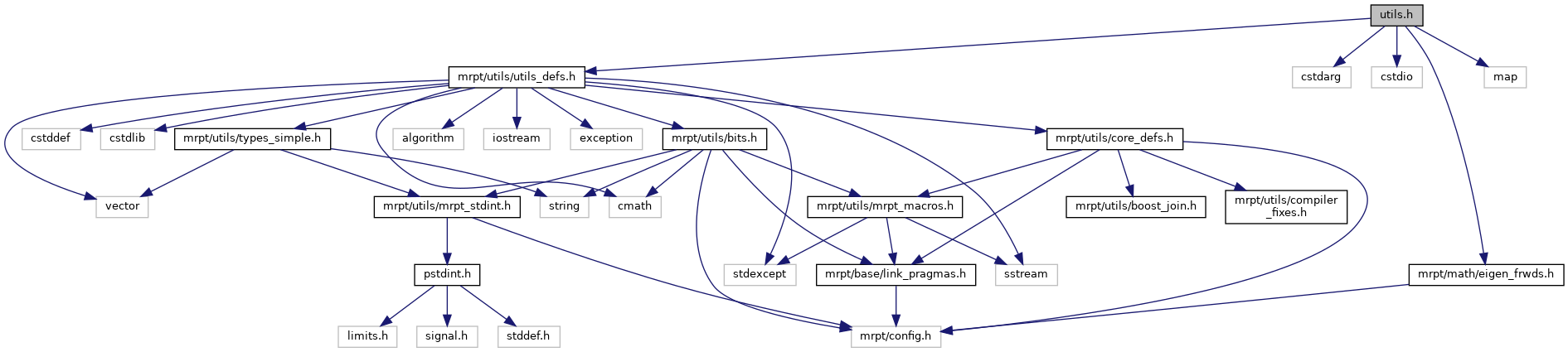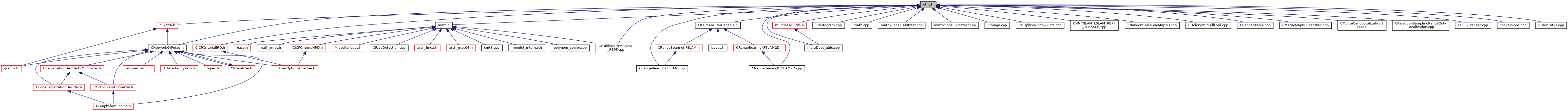Main MRPT website > C++ reference for MRPT 1.5.6
base/include/mrpt/math/utils.h File Reference
`#include <mrpt/utils/utils_defs.h>`
`#include <cstdarg>`
`#include <cstdio>`
`#include <mrpt/math/eigen_frwds.h>`
`#include <map>`
Include dependency graph for base/include/mrpt/math/utils.h:This graph shows which files directly or indirectly include this file:Go to the source code of this file.

Namespaces

mrpt
This is the global namespace for all Mobile Robot Programming Toolkit (MRPT) libraries.

mrpt::math
This base provides a set of functions for maths stuff.

Functions

template<class T1 , class T2 >
bool mrpt::math::approximatelyEqual (T1 a, T1 b, T2 epsilon)
Compare 2 floats and determine whether they are equal. More...

template<class T >
bool mrpt::math::approximatelyEqual (T a, T b)
Compare 2 floats and determine whether they are equal. More...

template<class T >
mrpt::math::absDiff (const T &lhs, const T &rhs)
Absolute difference between two numbers. More...

bool BASE_IMPEXP mrpt::math::loadVector (utils::CFileStream &f, std::vector< int > &d)
Loads one row of a text file as a numerical std::vector. More...

bool BASE_IMPEXP mrpt::math::loadVector (utils::CFileStream &f, std::vector< double > &d)
Loads one row of a text file as a numerical std::vector. More...

bool BASE_IMPEXP mrpt::math::isNaN (float f) MRPT_NO_THROWS
Returns true if the number is NaN. More...

bool BASE_IMPEXP mrpt::math::isNaN (double f) MRPT_NO_THROWS
Returns true if the number is NaN. More...

bool BASE_IMPEXP mrpt::math::isFinite (float f) MRPT_NO_THROWS
Returns true if the number is non infinity. More...

bool BASE_IMPEXP mrpt::math::isFinite (double f) MRPT_NO_THROWS
Returns true if the number is non infinity. More...

void BASE_IMPEXP mrpt::math::medianFilter (const std::vector< double > &inV, std::vector< double > &outV, const int &winSize, const int &numberOfSigmas=2)

template<typename T , typename VECTOR >
void mrpt::math::linspace (T first, T last, size_t count, VECTOR &out_vector)
Generates an equidistant sequence of numbers given the first one, the last one and the desired number of points. More...

template<class T , T STEP>
std::vector< T > mrpt::math::sequenceStdVec (T first, size_t length)
Generates a sequence of values [first,first+STEP,first+2*STEP,...]. More...

template<class VEC1 , class VEC2 >
void mrpt::math::normalize (const VEC1 &v, VEC2 &out_v)
Normalize a vector, such as its norm is the unity. More...

template<class VECTOR_OF_VECTORS , class VECTORLIKE >
void mrpt::math::extractColumnFromVectorOfVectors (const size_t colIndex, const VECTOR_OF_VECTORS &data, VECTORLIKE &out_column)
Extract a column from a vector of vectors, and store it in another vector. More...

uint64_t BASE_IMPEXP mrpt::math::factorial64 (unsigned int n)
Computes the factorial of an integer number and returns it as a 64-bit integer number. More...

double BASE_IMPEXP mrpt::math::factorial (unsigned int n)
Computes the factorial of an integer number and returns it as a double value (internally it uses logarithms for avoiding overflow). More...

template<class T >
mrpt::math::round2up (T val)
Round up to the nearest power of two of a given number. More...

std::string BASE_IMPEXP mrpt::math::MATLAB_plotCovariance2D (const CMatrixFloat &cov22, const CVectorFloat &mean, const float &stdCount, const std::string &style=std::string("b"), const size_t &nEllipsePoints=30)
Generates a string with the MATLAB commands required to plot an confidence interval (ellipse) for a 2D Gaussian ('float' version). More...

std::string BASE_IMPEXP mrpt::math::MATLAB_plotCovariance2D (const CMatrixDouble &cov22, const CVectorDouble &mean, const float &stdCount, const std::string &style=std::string("b"), const size_t &nEllipsePoints=30)
Generates a string with the MATLAB commands required to plot an confidence interval (ellipse) for a 2D Gaussian ('double' version). More...

template<typename EIGEN_VECTOR , typename At , size_t N>
EIGEN_VECTOR & mrpt::math::loadVector (EIGEN_VECTOR &v, At(&theArray)[N])
Assignment operator for initializing a std::vector from a C array (The vector will be automatically set to the correct size). More...

template<typename T , typename At , size_t N>
std::vector< T > & mrpt::math::loadVector (std::vector< T > &v, At(&theArray)[N])

template<size_t N, typename T >
std::vector< T > mrpt::math::make_vector (const T val1,...)
A versatile template to build vectors on-the-fly in a style close to MATLAB's v=[a b c d ...] The first argument of the template is the vector length, and the second the type of the numbers. More...

template<class TRIPLET >
bool mrpt::math::saveEigenSparseTripletsToFile (const std::string &sFile, std::vector< TRIPLET > &tri)
Saves to a plain-text file the nonzero entries of a Eigen sparse matrix, represented as a vector of triplets. More...

 Page generated by Doxygen 1.8.6 for MRPT 1.5.6 Git: 4c65e84 Tue Apr 24 08:18:17 2018 +0200 at mar abr 24 08:26:17 CEST 2018